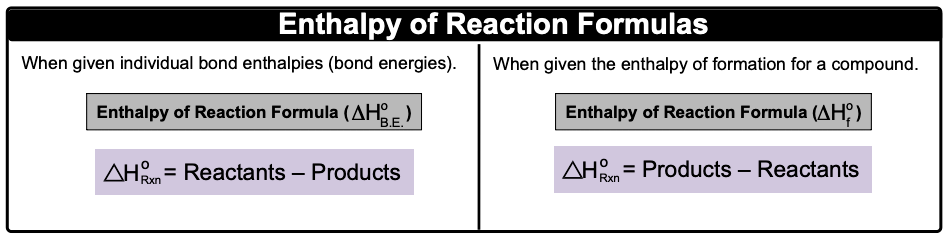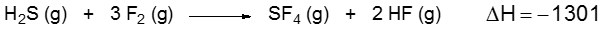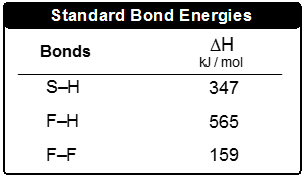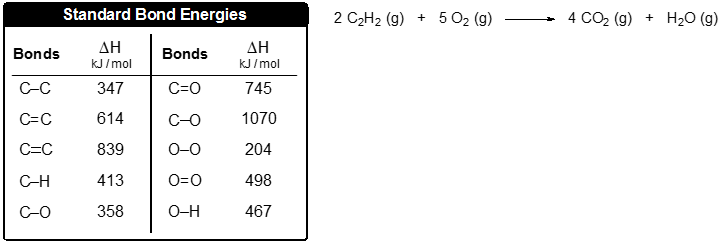Clutch Prep is now a part of Pearson
Ch.7 Energy, Rate and EquilibriumWorksheetSee all chapters

# Bond Energy

See all sections
Sections
Nature of Energy
First Law of Thermodynamics
Endothermic & Exothermic Reactions
Bond Energy
Thermochemical Equations
Heat Capacity
Thermal Equilibrium (Simplified)
Hess's Law
Rate of Reaction
Energy Diagrams
Chemical Equilibrium
The Equilibrium Constant
Le Chatelier's Principle
Solubility Product Constant (Ksp)
Spontaneous Reaction
Entropy (Simplified)
Gibbs Free Energy (Simplified)

Bond Energy is the amount of energy stored within a chemical bond.

###### Bond Energy

Concept #1: Bond Energy

When individual bond energies are given, we will use the first formula.Example #1: Calculate the ∆Horxn if the bond enthalpies of NΞN, H–H and N–H are 945 kJ/mol, 432 kJ/mol and 391 kJ/mol respectively.

Practice: Consider the following equation:Determine the bond enthalpy value for the F–S bond.Practice: Use the bond energies to estimate the enthalpy of reaction for the combustion of 5 moles of acetylene: